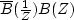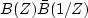Next: Spectral transfer function Up: CORRELATION AND SPECTRA Previous: Spectra of complex-valued signals

## Time-domain conjugate

A complex-valued signal

such ascan be imagined as a corkscrew, where the real and imaginary parts are plotted on the x- and y-axes, and time t runs down the axis of the screw. The complex conjugate of this signal reverses the y-axis and gives the screw an opposite handedness.  In Z-transform notation, the time-domain conjugate

is written(45)
Now consider the complex conjugate of a frequency function. In Z-transform notation this is written(46)
To see that it makes a difference in which domain we take a conjugate, contrast the two equations (45) and (46). The functionis a spectrum, whereas the functionis called an envelope function.''

For example, given complex-valued bt vanishing for t<0, the composite filteris a causal filter with a real time function, whereas the filteris noncausal and also a real-valued function of time. (The latter filter would turn out to be symmetric in time only if all bt were real.)

You might be tempted to think that,but that is true only ifis real, and often it is not. Chapteris largely devoted to exploring the meaning of complex frequency.Next: Spectral transfer function Up: CORRELATION AND SPECTRA Previous: Spectra of complex-valued signals
Stanford Exploration Project
10/21/1998